### Day 11 - Operations with Radicals - 09.02.14

 Bell Ringer Go over Quiz 2!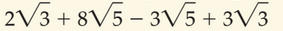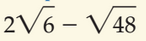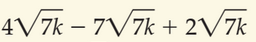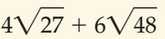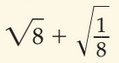Review Exponent Properties Product of Powers Power of a Power Power of a Product Quotient of Powers Power of a Quotient Zero Exponent Negative Exponents Radical Properties Product of Square Roots Quotient of Square Roots Rationalizing the Denominator Rational Exponents Exponential Growth and Decay Lesson Operations with RadicalsExtended Practicepage 595 #5-9 (odds)page 595 #15-29 (odds)Radical Equations Solve the following. Be sure to check for extraneous solutions.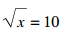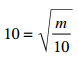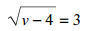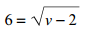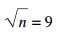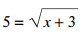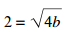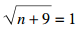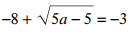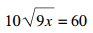Extended Practicepage 600 #5-13 (odds)page 601 #17-33 (odds)page 601 #35-47 (odds) Exit Ticket Will be posted on the board toward the end of the block... Lesson Objective(s) How are exponent properties applied to simplify problems? How are radical properties used to simplify radical expressions and solve radical equations? Standard(s) N.RN.1  Explain how the definition of the meaning of rational exponents follows from extending the properties of integer exponents to those values, allowing for a notation for radicals in terms of rational exponents. N.RN.2  Rewrite expressions involving radicals and rational exponents using the properties of exponents. A.CED.1 Create equations and inequalities in one variable and use them to solve problems. Include equations arising from linear and quadratic functions, and exponential functions. Mathematical Practice(s) #2: Reason abstractly and quantitatively Students will use concrete examples of numerical manipulation to examine closure of rational and irrational numbers. For example, students will use numeric examples of sums and products of rational numbers to generalize the closure of rational numbers under addition and multiplication. #4: Model with mathematics Students will create equations using rational or radical expressions to represent mathematical models of real-world situations like interest rates or depreciation.